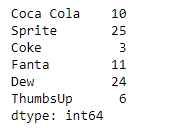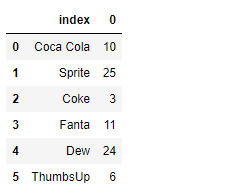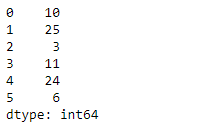Skip to content
Related Articles
Python | Pandas Series.reset_index()
• Last Updated : 10 Feb, 2019

Pandas series is a One-dimensional ndarray with axis labels. The labels need not be unique but must be a hashable type. The object supports both integer- and label-based indexing and provides a host of methods for performing operations involving the index.

Pandas` Series.reset_index()` function generate a new DataFrame or Series with the index reset. This comes handy when index is need to be used as a column.

Syntax: Series.reset_index(level=None, drop=False, name=None, inplace=False)

Parameter :
level : For a Series with a MultiIndex
drop : Just reset the index, without inserting it as a column in the new DataFrame.
name : The name to use for the column containing the original Series values.
inplace : Modify the Series in place

Returns : result : Series

Example #1: Use `Series.reset_index()` function to reset the index of the given Series object.

 `# importing pandas as pd``import` `pandas as pd`` ` `# Creating the Series``sr ``=` `pd.Series([``10``, ``25``, ``3``, ``11``, ``24``, ``6``])`` ` `# Create the Index``index_ ``=` `[``'Coca Cola'``, ``'Sprite'``, ``'Coke'``, ``'Fanta'``, ``'Dew'``, ``'ThumbsUp'``]`` ` `# set the index``sr.index ``=` `index_`` ` `# Print the series``print``(sr)`

Output :Now we will use `Series.reset_index()` function to reset the index of the given series object.

 `# reset the index``result ``=` `sr.reset_index()`` ` `# Print the result``print``(result)`

Output :As we can see in the output, the `Series.reset_index()` function has reset the index of the given Series object to default. It has preserved the index and it has converted it to a column.

Example #2: Use `Series.reset_index()` function to reset the index of the given Series object. Do not keep the original index labels of the given series object.

 `# importing pandas as pd``import` `pandas as pd`` ` `# Creating the Series``sr ``=` `pd.Series([``10``, ``25``, ``3``, ``11``, ``24``, ``6``])`` ` `# Create the Index``index_ ``=` `[``'Coca Cola'``, ``'Sprite'``, ``'Coke'``, ``'Fanta'``, ``'Dew'``, ``'ThumbsUp'``]`` ` `# set the index``sr.index ``=` `index_`` ` `# Print the series``print``(sr)`

Output :Now we will use `Series.reset_index()` function to reset the index of the given series object and also we will be dropping the original index labels.

 `# reset the index``result ``=` `sr.reset_index(drop ``=` `True``)`` ` `# Print the result``print``(result)`

Output :As we can see in the output, the `Series.reset_index()` function has reset the index of the given Series object to default. It has dropped the original index.

Attention geek! Strengthen your foundations with the Python Programming Foundation Course and learn the basics.

To begin with, your interview preparations Enhance your Data Structures concepts with the Python DS Course. And to begin with your Machine Learning Journey, join the Machine Learning – Basic Level Course

My Personal Notes arrow_drop_up